April 24, 2019

How to build a Convolutional Neural Network with Keras

In this tutorial I will use Keras to build a convolutional neural network (CNN) to classify Tesla cars.

I already explain the magic behind this neural networks in this post. Therefore, I will not explain how they work.

Keras and more libraries

We will need some Keras and some more libraries to build our neural network, this is a link of the notebook with all the code of this tutorial.

import numpy as np
import os
import shutil
from keras.utils import to_categorical

from keras.models import Sequential
from keras.layers import Dense, Flatten, Activation, regularizers
from keras.layers import Conv2D, MaxPooling2D
from keras import optimizers

from keras.preprocessing.image import ImageDataGenerator, array_to_img, img_to_array, load_img
from keras.callbacks import ModelCheckpoint

from matplotlib import pyplot as plt
import os

As we have previously seen in the neural networks tutorial, first we need to download the images from GitHub:

wget https://github.com/vincent1bt/tesla-cars-dataset/archive/master.zip # descargar imagenes

unzip -qq master.zip
mkdir -p validation_images/tesla_model_3 && mkdir validation_images/tesla_model_s && mkdir validation_images/tesla_model_x # crear carpetas de validacion

Then we build the validation set:

validation_set_size = 30

def move_images(from_path, to_path):
files = os.listdir(from_path)
folder_size = len(files)
first_index = folder_size - validation_set_size
files_to_move = files[first_index:]

for file_name in files_to_move:
source_file_name = from_path + file_name
destination_file_name = to_path + file_name
shutil.move(source_file_name, destination_file_name)

move_images("./tesla-cars-dataset-master/tesla-model-3/", "./validation_images/tesla_model_3/")
move_images("./tesla-cars-dataset-master/tesla-model-s/", "./validation_images/tesla_model_s/")
move_images("./tesla-cars-dataset-master/tesla-model-x/", "./validation_images/tesla_model_x/")

We rename the folders:

mv tesla-cars-dataset-master training_images
mv training_images/tesla-model-3 training_images/tesla_model_3
mv training_images/tesla-model-s training_images/tesla_model_s
mv training_images/tesla-model-x training_images/tesla_model_x

Now we create the X variable that contains the images and the y variable that contains the labels:

img_height = 256
img_width = 256

X = []
y = []

for path in paths:
images_paths = os.listdir(path)

for image_path in images_paths:
complete_path = path + image_path
image_array = img_to_array(image)
X.append(image_array)
label = paths.index(path)
y.append(label)

return X, y

training_paths = ["training_images/tesla_model_3/", "training_images/tesla_model_s/", "training_images/tesla_model_x/"]
validation_paths = ["validation_images/tesla_model_3/", "validation_images/tesla_model_s/", "validation_images/tesla_model_x/"]

We convert the variables to numpy arrays:

X_train = np.array(X_train)
X_val = np.array(X_val)

y_train = np.array(y_train)
y_val = np.array(y_val)

As we know, Keras needs a different format for the y variable, this format is called one-hot-encode:

y_train = to_categorical(y_train)
y_val = to_categorical(y_val)

Data Augmentation

Now we are going to use data augmentation in order to obtain more images, if we have one image like the following one: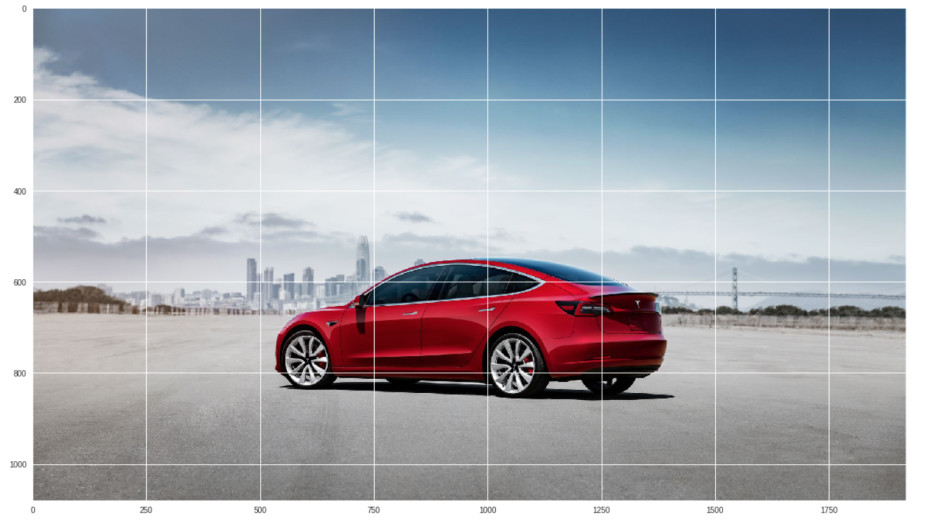This method will apply some random transformations to generate more images: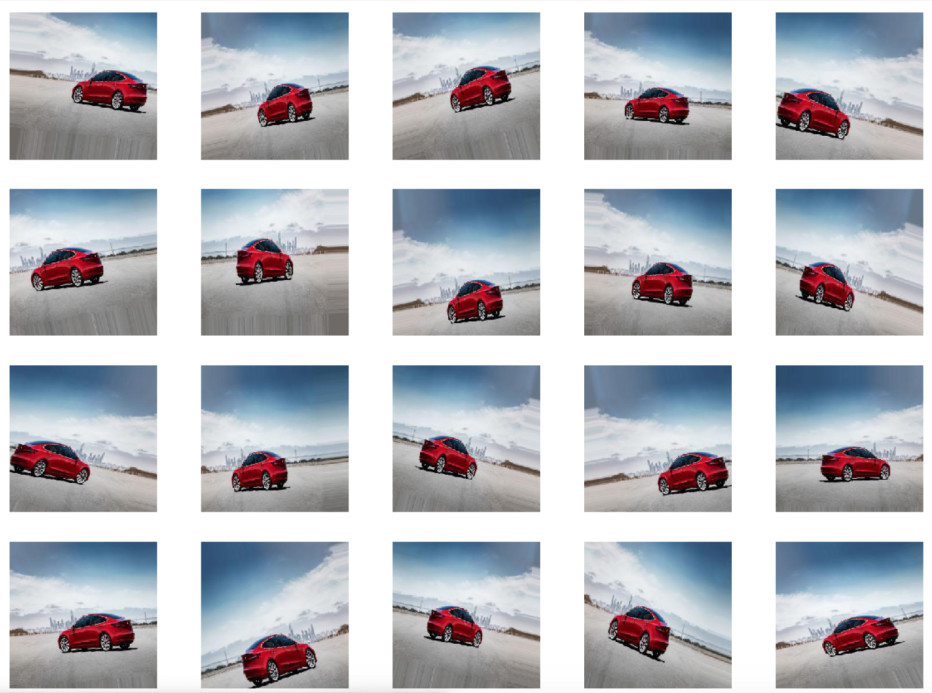These transformations can be done by the ImageDataGenerator Keras function:

datagen = ImageDataGenerator(
rotation_range = 20,
width_shift_range = 0.2,
height_shift_range = 0.2,
rescale = 1. / 255,
shear_range = 0.2,
zoom_range = 0.2,
horizontal_flip = True,
fill_mode = 'nearest')

This method has some parameters to apply different transformations to the image:

• rotation_range: the range of rotations the function will apply to the image.
• width shift range, height shift range: this changes the pixels’ orientation of some areas of the image.
• rescale: rescale the image from 0-255 to 0-1.
• shear_range: shear the image some degrees.
• zoom_range: Applies a random zoom to an area of the image
• horizontal_flip: flips the orientation of the image horizontally
• fill_mode: some transformations cut areas of the image, therefore we need to fill those missing pixeles, the nearest value uses the near pixels to fill these areas.

We will use this method to see some transformations, first we need a new folder called preview:

mkdir preview

We need to load an image:

img = img_to_array(img)
img = img.reshape((1,) + img.shape)

i = 0
for batch in datagen.flow(img, batch_size=1,
save_to_dir='preview', save_prefix='car', save_format='jpeg'):
i += 1
if i > 20:
break

With the code above we created 20 new images with transformations and we saved them in the preview folder.

Now we can see these images:

path = "./preview/"
X = []

images_paths = os.listdir(path)

for image_path in images_paths:
complete_path = path + image_path
X.append(image)

return X

def plot_images(images):
fig, axes = plt.subplots(4, 5)
plt.rcParams["figure.figsize"] = (20, 15)

for i, ax in enumerate(axes.flat):
ax.imshow(images[i])

ax.set_xticks([])
ax.set_yticks([])

plt.show()

plot_images(X_preview)

We will use two different generators, one for the training set where we want to generate more images and one for the validation set where we only want to rescale the current images:

train_generator = ImageDataGenerator(
rotation_range = 20,
width_shift_range = 0.2,
height_shift_range = 0.2,
rescale = 1. / 255,
shear_range = 0.2,
zoom_range = 0.2,
horizontal_flip = True,
fill_mode = 'nearest')

valid_generator = ImageDataGenerator(rescale = 1. / 255)

Building the model

Our images have a size of 256x256x3 and all the convolutional layers will use kernels of size 5x5.

input_shape=(256, 256, 3)
kernel_size = 5

The function below will plot the loss and the accuracy values obtained in each epoch:

def plot_loss_and_accuracy(model_trained):
accuracy = model_trained.history['acc']
val_accuracy = model_trained.history['val_acc']
loss = model_trained.history['loss']
val_loss = model_trained.history['val_loss']
epochs = range(len(accuracy))
plt.plot(epochs, accuracy, 'b', label='Training accuracy')
plt.plot(epochs, val_accuracy, 'r', label='Validation accuracy')
plt.ylim(ymin=0)
plt.ylim(ymax=1)
plt.xlabel('Epochs ', fontsize=16)
plt.ylabel('Accuracity', fontsize=16)
plt.title('Training and validation accuracy', fontsize = 20)
plt.legend()
plt.figure()
plt.plot(epochs, loss, 'b', label='Training loss')
plt.plot(epochs, val_loss, 'r', label='Validation loss')
plt.xlabel('Epochs ', fontsize=16)
plt.ylabel('Loss', fontsize=16)
plt.title('Training and validation loss', fontsize= 20)
plt.legend()
plt.show()

The following function will create, train and return the trained model:

def create_model(X_train, X_val, y_train, y_val, learning_rate, epochs, batch_size, callbacks):
model = Sequential()

#First layer
strides=1, input_shape=input_shape))

#Second layer

#Third layer
strides=1,))

#Fourth layer

#Classification

model_trained = model.fit_generator(train_generator.flow(X_train, y_train, batch_size=batch_size, shuffle = True), steps_per_epoch=len(X_train) // batch_size, epochs=epochs, verbose=1, callbacks=callbacks, validation_data=valid_generator.flow(X_val, y_val, shuffle = True), validation_steps=len(X_val) // batch_size)

return model_trained, model

This model has:

• 3 convolutional layers with 64 kernels of size 5x5 each one, no padding and a stride value of 1.
• 3 pooling layers with the default parameters values.
• 1 dense layer with 500 neurons.
• 1 output/dense layer with 3 neurons for the 3 classes.

All the layers has the activation function relu except for the output layer, in this last layer we used the activation function softmax, also we used the Adam optimizer with a learning rate of 0.0003.

The fit_generator method trains the neural network with the generators we previously created, when we use the fit_generator method the new images are created when the model needs them and then are deleted, with this we don’t have to use a lot of memory to store these images.

When we are using generators we need to specify two new parameters called steps_per_epoch and validation_steps, since the generators run indefinitely, with these parameters we tell them when they have to stop, if we have 320 images and a batch_size of 32, then we have 10 steps per epoch, in other words, we need 10 steps in each epoch to see all the images available.

Finally we have one last parameter called callbacks, this parameter executes one or several functions after each epoch, Keras already has some functions to be used as callbacks, in this model we used the callback ModelCheckpoint, this callback saves the weights values (kernels) each time the model improves the accuracy or loss value.

Now we define the hyperparameters for this model and the callback function:

epochs = 250
batch_size = 32
learning_rate = 0.0003

callbacks = [ModelCheckpoint(filepath='weights.{epoch:02d}-val_acc:{val_acc:.2f}.h5', monitor='val_acc', save_best_only=True, verbose=1)]

We train the model and plot the loss and the accuracy values:

model_trained, model = create_model(X_train, X_val, y_train, y_val, learning_rate, epochs, batch_size, callbacks)

plot_loss_and_accuracy(model_trained)
validation_acc = model_trained.history['val_acc'][-1] * 100
training_acc = model_trained.history['acc'][-1] * 100
print("Validation accuracy: {}%\nTraining Accuracy: {}%".format(validation_acc, training_acc))

We can reach an accuracy of 84% in the validation set, this is a good value taking into account that we only have 446 images.

As we saw in the previous section, the callback ModelCheckpoint saves the best weights values, we can load these weights to the same model or create a new model:

def create_empty_model(learning_rate):
model = Sequential()

strides=1, input_shape=input_shape))

strides=1,))

return model

best_model = create_empty_model(learning_rate)

Using the model to make predictions

We are going to test the model with 3 new images, one image for each class:

wget https://static.urbantecno.com/2018/08/Tesla-Model-3-4-720x550.jpg

X_test = []

image_array = img_to_array(image)
X_test.append(image_array)

image_array = img_to_array(image)
X_test.append(image_array)

image_array = img_to_array(image)
X_test.append(image_array)

X_test = np.array(X_test)

X_test = X_test.astype('float32') / 255

We run the model's predict function:

y_pred = best_model.predict(X_test, batch_size=None, verbose=1, steps=None)

The predicted labels (y_pred) should match the true labels (y_true):

np.argmax(y_true, axis=1), np.argmax(y_pred, axis=1)
(array([0, 1, 2]), array([1, 1, 2]))

Even though the model predicted correctly 2 of 3 classes, this is a good result, after all we have a small dataset, we can also see that the model predicted a model 3 car as a model s and these cars are similar.

Checking more predictions

We can see the predictions that the model made and the true classes for some images:

img_height = 256
img_width = 256

X = []
y = []

for path in paths:
images_paths = os.listdir(path)

for image_path in images_paths:
complete_path = path + image_path
X.append(image)
label = paths.index(path)
y.append(label)

return X, y

X_train = X_train.astype('float32') / 255
X_val = X_val.astype('float32') / 255

y_train_pred = best_model.predict(X_train, batch_size=None, verbose=1, steps=None)
y_val_pred = best_model.predict(X_val, batch_size=None, verbose=1, steps=None)

y_train_pred_max = np.argmax(y_train_pred, axis=1)
y_val_pred_max = np.argmax(y_val_pred, axis=1)

y_train_true = np.argmax(y_train, axis=1)
y_val_true = np.argmax(y_val, axis=1)

With the code below we can print each image, its real class and the class predicted by the model:

classes_array = ['3', 's', 'x']

def plot_images(images, cls_true, cls_pred):
fig, axes = plt.subplots(8, 8)
plt.rcParams["figure.figsize"] = (20, 20)

for i, ax in enumerate(axes.flat):
ax.imshow(images[i])

true_class = classes_array[int(cls_true[i])]
pred_class = classes_array[int(cls_pred[i])]

xlabel = "True: {0}, Pred: {1}".format(true_class, pred_class)

ax.set_xlabel(xlabel)

ax.set_xticks([])
ax.set_yticks([])

plt.show()

We will use 64 images of the first class (tesla model 3):

images = X_complete[0:64]
cls_true = y_train_true[0:64]
cls_pred = y_train_pred_max[0:64]
plot_images(images, cls_true, cls_pred)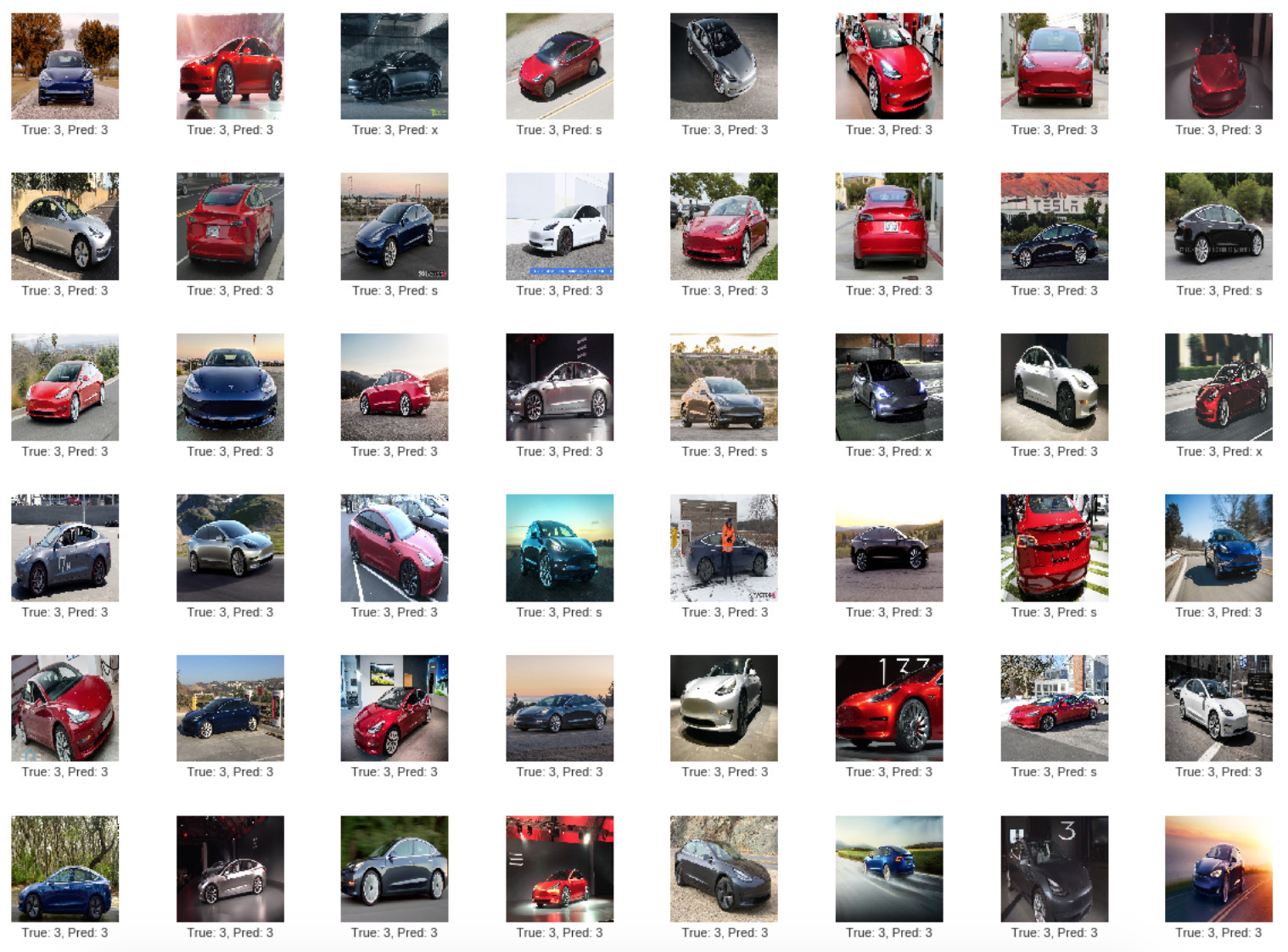We can see that when the car is black the model thinks it is a Tesla model x.

We will use 64 images of the second class (tesla model s) as well:

images = X_complete[150:214]
cls_true = y_train_true[150:214]
cls_pred = y_train_pred_max[150:214]
plot_images(images, cls_true, cls_pred)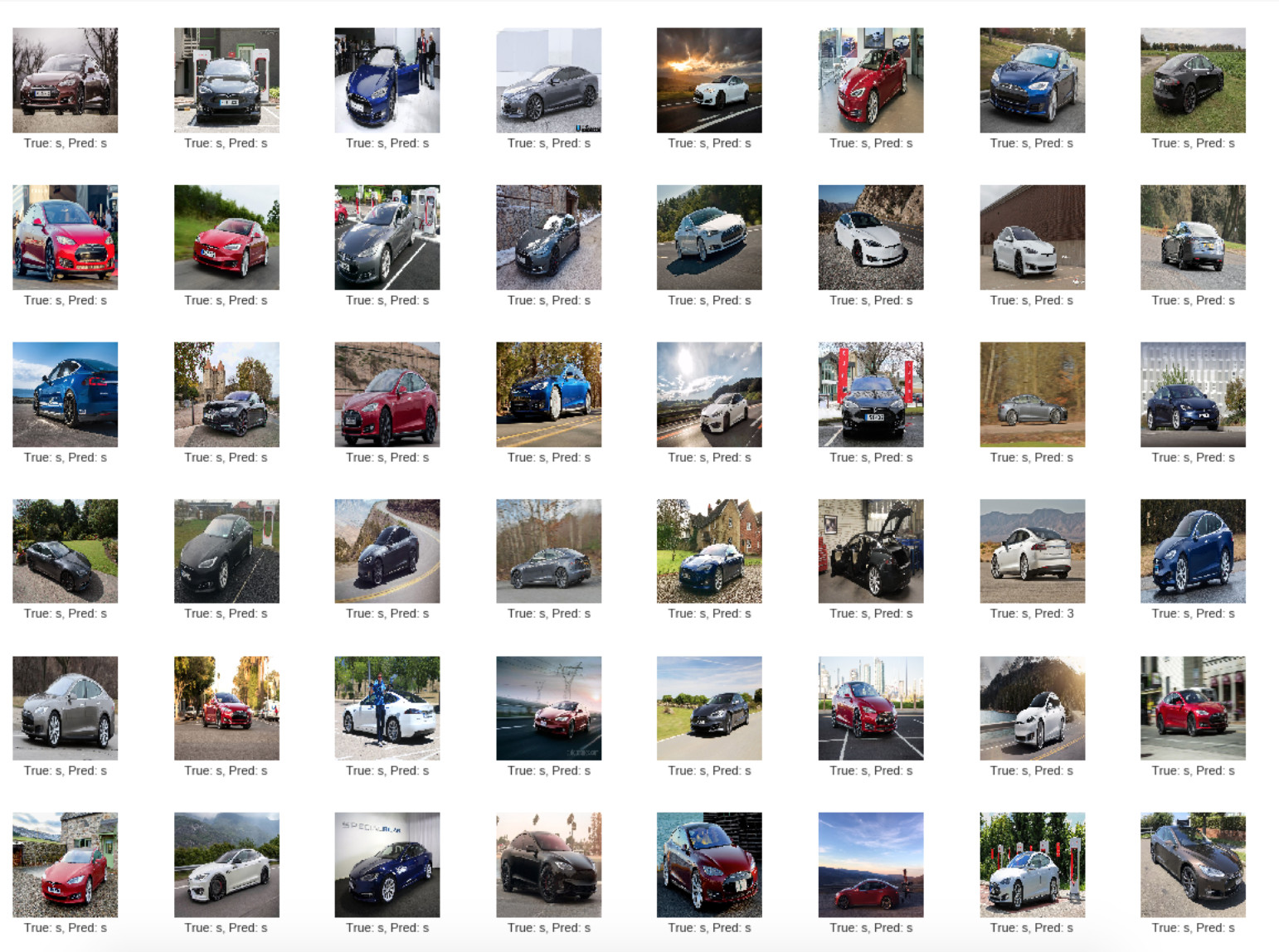You can see more information in the notebook with all the code

We can also print the kernels:

total_kernel = 64
conv1_kernels = best_model.layers.get_weights()
plt.rcParams["figure.figsize"] = (15, 15)

for i in range(total_kernel):
plt.subplot(8, 8, i + 1)
plt.imshow(conv1_kernels[:, :, 0, i], cmap='BrBG')
plt.axis('off')

These are the 64 kernels of the first convolutional layer: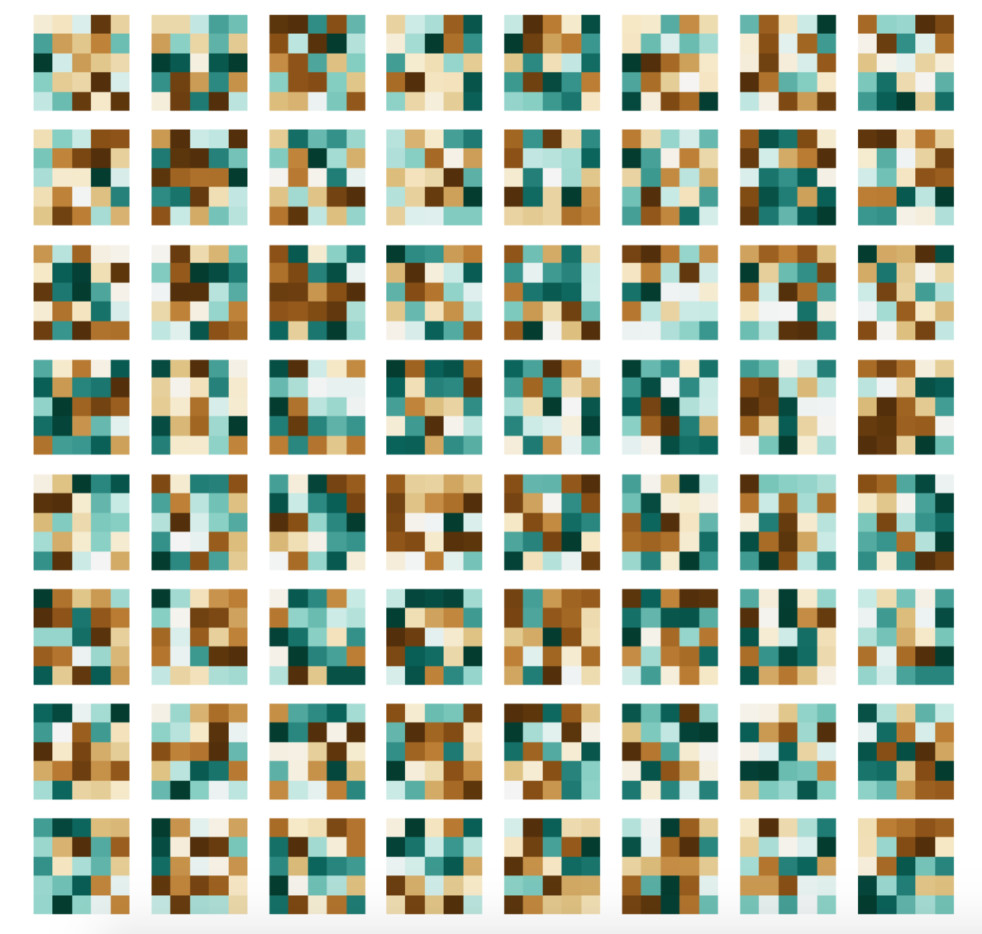Each kernel has a size of 5x5.